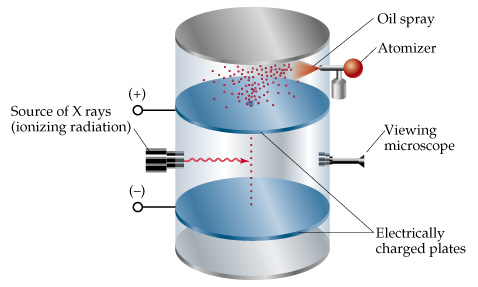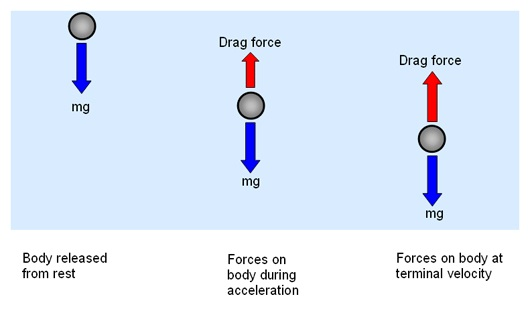#A Historical Experiment

In 1909, physics pioneers Robert Millikan (1868-1953) and Harvey Fletcher (1884-1981) performed an experiment that would ultimately enable them to determine one of the most fundamental of all physical constants: the elementary electric charge, i.e. the electric charge of an individual electron – the constant e

What Millikan and Fletcher did in their famous experiment, was to charge electrically a tiny drop of oil, then measure precisely how strong an applied electric field had to be in order to stop the oil drop from falling.  Since they were able to work out the mass of the oil drop, they could subsequently calculate the force of gravity on one drop, and then determine the electric charge that the drop must have.

By varying the charge on different drops, the pair noticed that the electrical charge was always consistently a multiple of – 1.6 x 10-19 C (or Coulomb), the charge on a single electron.  This meant that it was electrons carrying this unit charge e.

Repeating the Millikan experiment over and over for many droplets, they confirmed that the charges were indeed all multiples of some fundamental value of charge.  They calculated it to be exactly 1.5924(17) x 10-19 C.

Their value was within 1% of the currently accepted value of

### e = – 1.602176487(40) x 10-19 C

They proposed this to be the charge e on a single electron.

# Balancing Forces in Mechanical Equilibrium

consisted in balancing the downward gravitational force, with the upward drag and electric forces on tiny charged droplets of oil suspended between two metal electrodes.  Since the density of the oil was known, the droplets’ masses, and their gravitational and buoyant forces, could be determined from their observed radii.  Using a known electric field, Millikan and Fletcher managed to determine the charge on oil droplets in mechanical equilibrium.

# Millikan Experimental Setup

A fine mist of oil droplets was sprayed into a chamber above the plates. The oil was of a type usually used in vacuum apparatus, and chosen because it had an extremely low vapour pressure.  Ordinary oil would evaporate under the heat of the light source, causing the mass of the oil drop to change over the course of the experiment.

Some oil droplets became electrically charged through simple friction with the nozzle as they were sprayed.  Alternatively, charging could be brought about by including an ionising radiation source (such as an X-ray tube).  The droplets entered the space between the plates and, because they were charged, it could be made to rise and fall by changing the voltage across the plates.

#The Scientific Method

Initially, the oil drops are allowed to fall between the plates with the electric field turned off.  The droplets very quickly reach a terminal velocity, because of friction with the air in the chamber.

The electric field is then turned on and, if it is large enough, the charged drops start to rise.

The reason for this is because the upwards electric force FE is greater for them than the downwards gravitational force Fg.  (In the same way, bits of paper can be picked up by a charged plastic ruler).

A likely looking oil droplet is then selected, and maintained in the middle of the field of view by alternately switching off the voltage, until all the other drops have fallen.  The Millikan experiment is then continued with this one drop.

The drop is allowed to fall and its terminal velocity v1 (i.e. the velocity in the absence of an electric field), is calculated.

Let’s see how…

# Using Stokes’ Law of Fluid Mechanics

In 1851, mathematical physicist George Stokes derived an expression for the frictional force (or drag force) exerted on spherical objects with very small ‘Reynolds numbers’ (very small particles) in a continuous viscous fluid.  Stokes’ equation can be used in modelling the settlement of fine particles in water or other fluids.

In air, Stokes’ Law explains why small water droplets (or ice crystals) can remain suspended in air (as clouds) until they grow to a critical size, then start falling as rain, or hail, or snow…

Stokes’ Drag, Fd, the frictional force acting on the oil droplet, is calculated with Stokes’ law:$\boldsymbol {F_d} = 6 \pi r \eta \boldsymbol {v_1}$

where v1 is the terminal velocity (i.e. the velocity in the absence of an electric field) of the falling drop, η is the air viscosity, and r is the radius of the actual drop.The weight w is the mass m of the particle multiplied by the acceleration due to gravity g. It is also given by multiplying the volume D by the density ρ and the acceleration due to gravity g.

However, what is needed for the Millikan experiment is the apparent weight.

The apparent weight in air ω is the true weight minus the up-thrust (which equals the weight of air displaced by the oil drop).

For a perfectly spherical droplet, the apparent weight can be written as:$\boldsymbol \omega = \frac {4 \pi}{3} r^3 (\rho - \rho_{air}) \boldsymbol g$

At terminal velocity, the oil drop is no longer accelerating.

At this point in time, the total force acting on it must be 0, and the two forces F and w must cancel each other out.

That is,$\boldsymbol F_d = \boldsymbol \omega$

This implies$r^2 = \frac {9 \eta \boldsymbol v_1}{2 \boldsymbol g (\rho - \rho_{air})}$

Once r is calculated, w can be worked out.

# Resuming The Millikan Experiment

Now the field is turned back on, and the electric force on the drop is$\boldsymbol F_E = q \boldsymbol E$

where q is the charge on the oil drop and E is the electric field between the plates.

For parallel plates, we have$\boldsymbol E = \frac {\boldsymbol V}{d}$

where V is the voltage (or potential difference) and d is the distance between the plates.

One way to determine the elementary charge q in the Millikan experiment, is to adjust the voltage V until the oil drop remained steady.  Then, we can equate FE with w.  Also, determining FE proves difficult because the mass of the oil drop is tricky to determine without reverting to the use of Stokes’ Law.

A more practical approach consists in turn V up slightly, so that the oil drop rises with a new terminal velocity v2.

Subsequently,$q \boldsymbol E - \boldsymbol w = 6 \pi \eta (\boldsymbol {r \centerdot v_2}) = \lvert \frac {\boldsymbol v_2}{\boldsymbol v_1} \rvert \boldsymbol w$

And the elementary charge e?

The experiment proved that the charge on the oil drop changed always by a quantity ne where n is chosen to be a positive or negative integer.  Using different kinds of drops in different mediums, Millikan determined that the dependence was always the same$e \propto \frac {mg}{E n} (v_2 - v_1)$

The currently accepted value for the elementary charge is

###$e = -1.602176487(40) \times 10^{-19}C$$e = -1.602176487(40) \times 10^{-19}C$

But actually, that’s not entirely true.  Well, okay, it is, but everything is moving so fast in Physics…

The elementary charge on an electron (or for that matter on a proton) does not really stand up well as the ‘elementary’ charge any longer.

What we are now beginning to know as the elementary charge is the “quantum of charge“.  And that’s$\frac {1}{3} \boldsymbol e$.

Enough material there for another post, I think so…  Bear with!)

# The Millikan Experiment as an Example of Psychological Effects in Scientific Methodology

In 1974, physicist Richard Feynman noted on the subject of the Millikan experiment:

“We have learned a lot from experience about how to handle some of the ways we fool ourselves.  One example: Millikan measured the charge on an electron by an experiment with falling oil drops, and got an answer which we now know not to be quite right. It’s a little bit off because he had the incorrect value for the viscosity of air.  It’s interesting to look at the history of measurements of the charge of an electron, after Millikan.  If you plot them as a function of time, you find that one is a little bit bigger than Millikan’s, and the next one’s a little bit bigger than that, and the next one’s a little bit bigger than that, until finally they settle down to a number which is higher.Why didn’t they discover the new number was higher right away?  It’s a thing that scientists are ashamed of – this history – because it’s apparent that people did things like this: When they got a number that was too high above Millikan’s, they thought something must be wrong – and they would look for and find a reason why something might be wrong.  When they got a number close to Millikan’s value they didn’t look so hard.  And so they eliminated the numbers that were too far off, and did other things like that…”

Surely you’re joking, Mr Feynman!

# Calling It The Observer BiasThe observer bias is the well-known tendency for a researcher to draw conclusions from a set of evidence based on his or her own preconceptions or understanding of the problem.

It is a clear manifestation of the inevitable trumping of the idealised objectivity of science by human nature.

The historical Millikan experiment, carried out to determine the measurement of the charge on an individual electron, which tells us one of the most basic constants in physics, is a well-known example of this.  And although Millikan’s exclusions of data did not affect his final value of e, there is no doubt regarding the fact that Millikan did perform substantial “cosmetic surgery” on his data, which had the effect of reducing the statistical error on e.

This enabled Millikan to claim that he had calculated e to better than one half of a percent.  If Millikan had included all of the data that he selectively discarded, his result would have been accurate to within 2%.

While this would still have resulted in Millikan having measured the elementary charge e better than anyone else at the time, the larger uncertainty might have allowed more scientists within the Physics community to challenge the accuracy of his findings.